# Prometheus 四种metric类型

Prometheus的4种metrics（指标）类型：

• Counter
• Gauge
• Histogram
• Summary

#### Counter 与 Gauge

##### Counter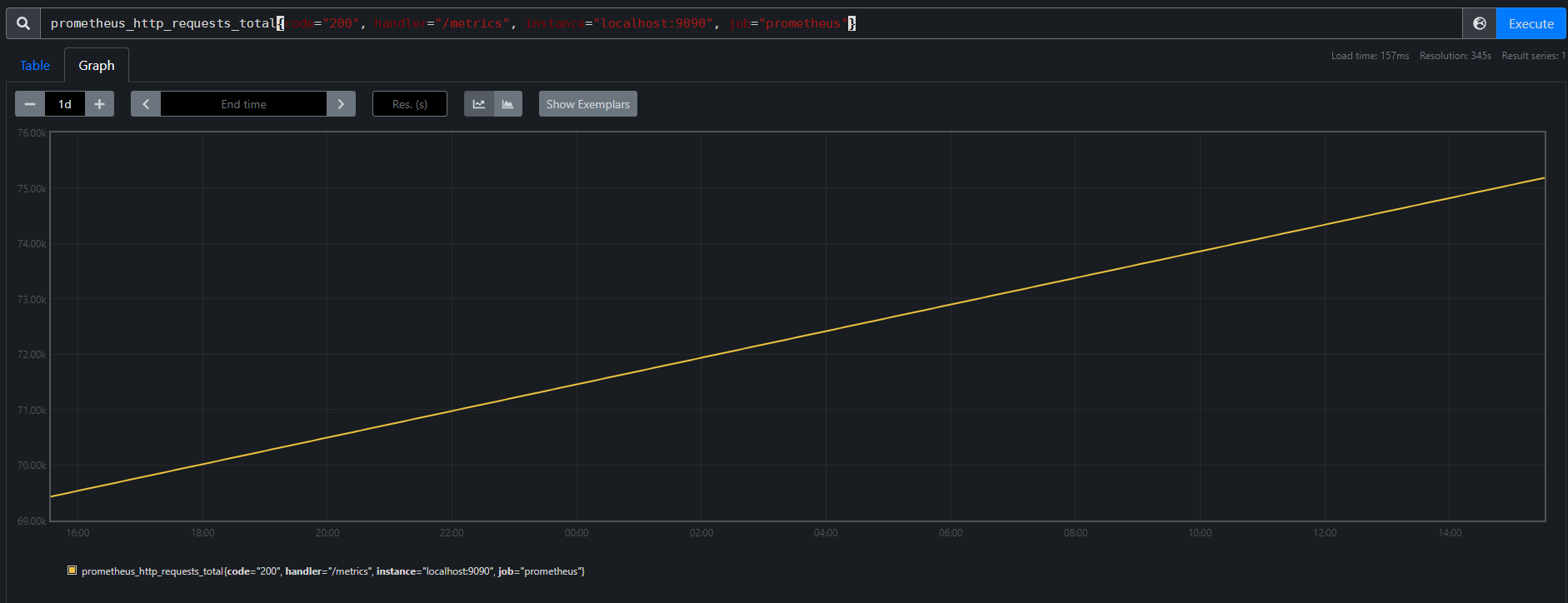##### Gauge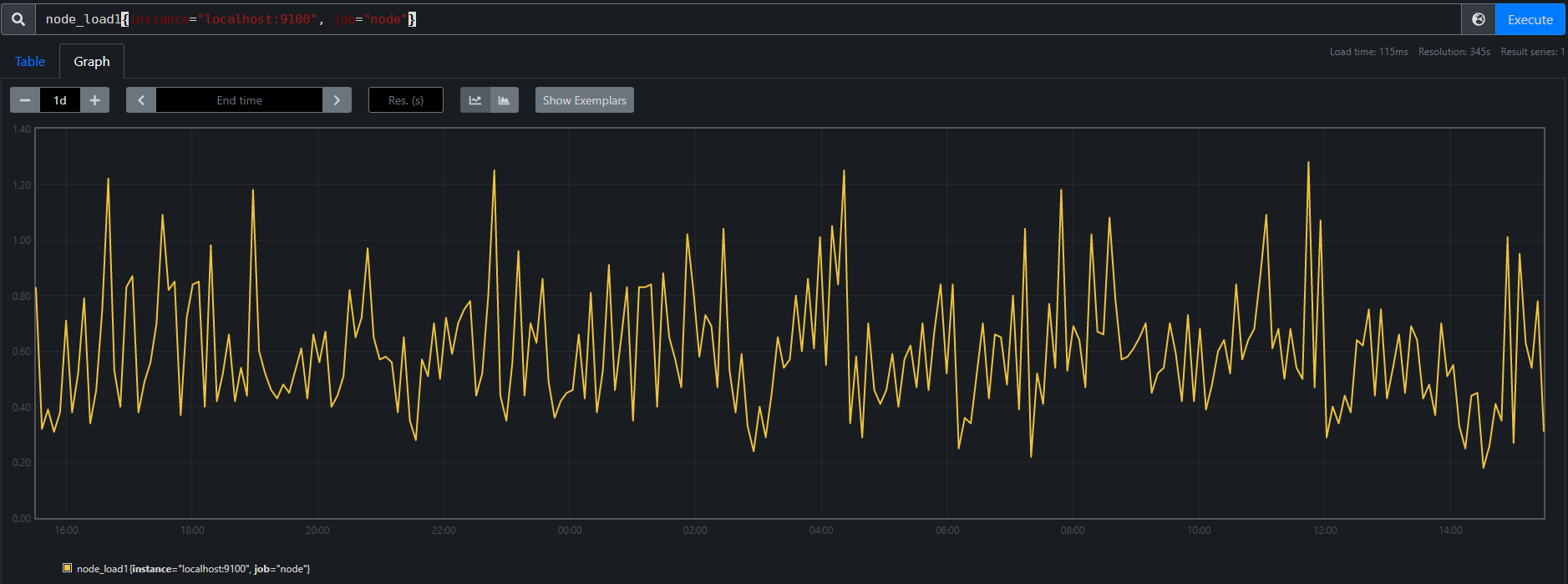#### Histogram 与 Summary

##### Histogram

• 桶累积计数器，<basename>_bucket{le="<upper inclusive bound>"}
• 所有观察值的总和，<basename>_sum
• 已观察到的事件计数，<basename>_count与上述相同<basename>_bucket{le="+Inf"}

kubelet_pod_start_duration_seconds_bucket
kubelet_pod_start_duration_seconds_count #进行过pod start的数量
kubelet_pod_start_duration_seconds_sum  # 总耗时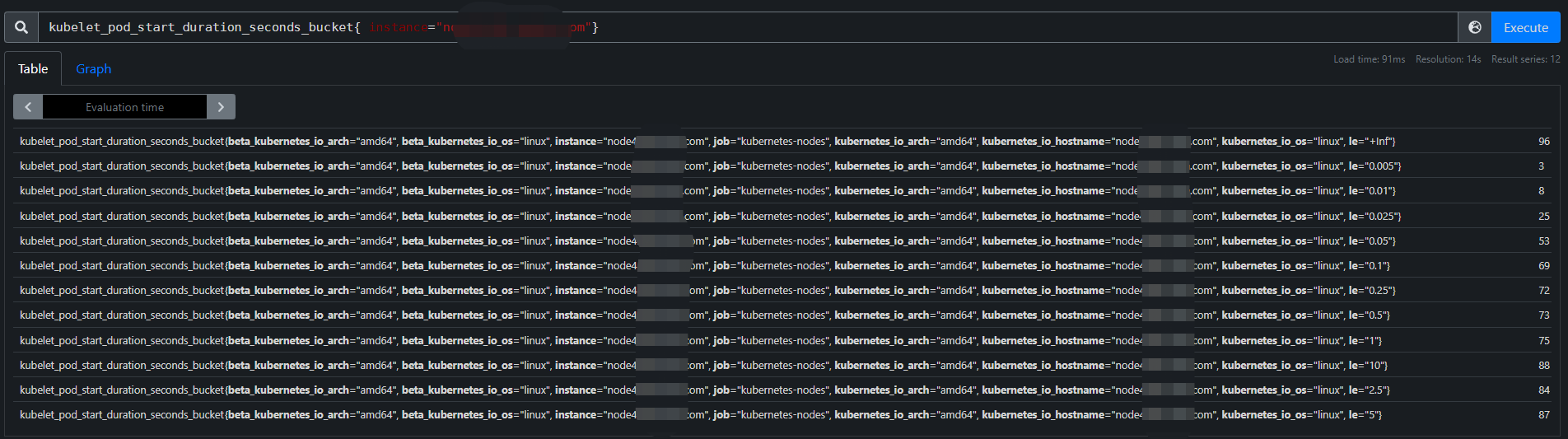le:小于等于。例如le=”5“，即pod启动耗时<=5s的有87次

+inf：无穷。当启动耗时为无穷时，也就是节点下pod启动过的数量，与kubelet_pod_start_duration_seconds_count相等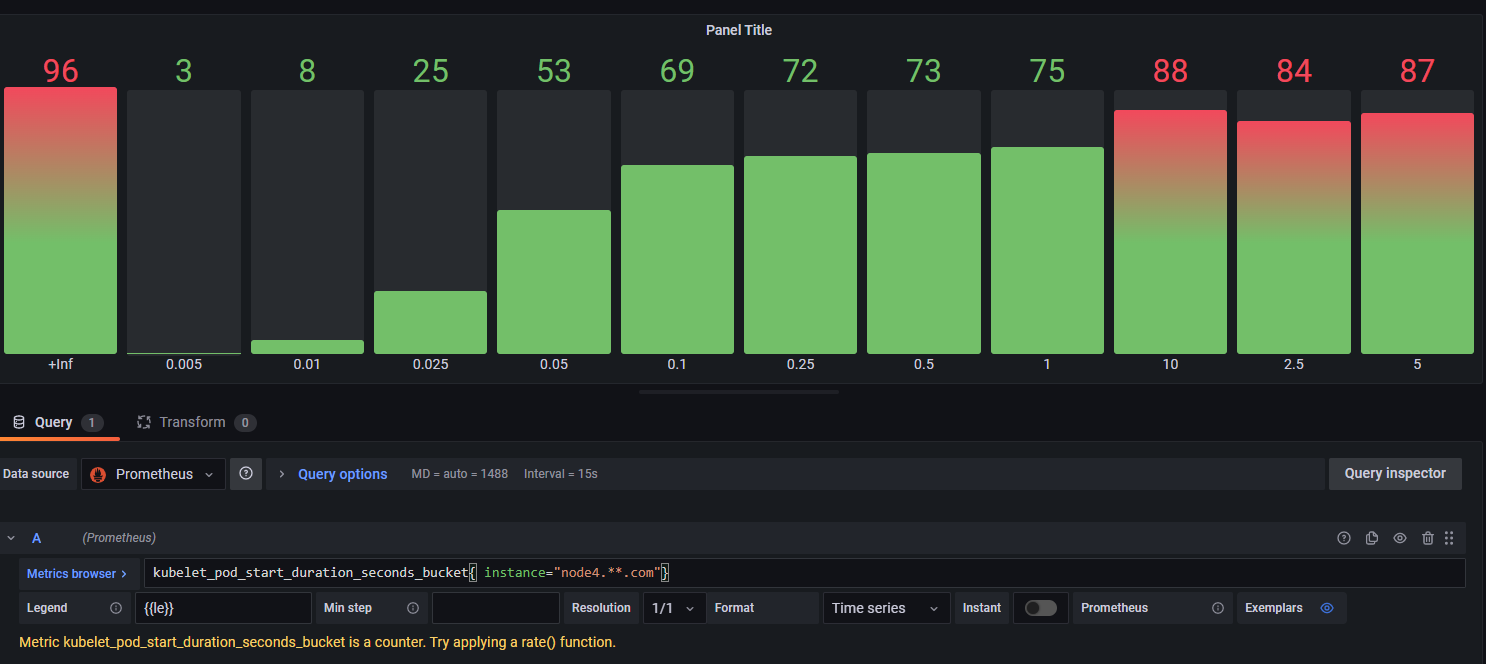#kubelet_pod_start_duration_seconds_bucket{ instance="node4.**.com",le="2.5"} - ignoring(le) kubelet_pod_start_duration_seconds_bucket{ instance="node4.**.com",le="1"}
9


#kubelet_pod_start_duration_seconds_bucket{ instance="node4.**.com",le="2.5"} / ignoring(le) kubelet_pod_start_duration_seconds_bucket{ instance="node4.**.com",le="+Inf"}
0.875


#histogram_quantile(0.80,kubelet_pod_start_duration_seconds_bucket{ instance="node4.**.com"} )
1.3000000000000018


histogram_quantile(0.8, sum(rate(prometheus_http_request_duration_seconds_bucket[5m])) by (le))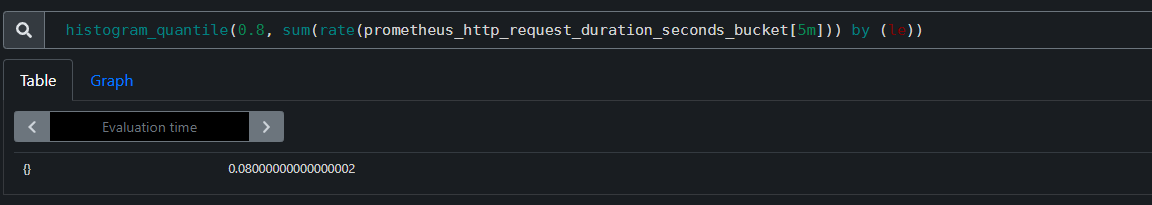Apdex 指数 =( 满意数量 + 0.5 * 可容忍数量 ) / 总样本数，假设请求满意时间为0.3s，则可容忍时间为1.2s（4倍）

(
sum(rate(http_request_duration_seconds_bucket{le="0.3"}[5m])) by (job)
+
sum(rate(http_request_duration_seconds_bucket{le="1.2"}[5m])) by (job)
) / 2 / sum(rate(http_request_duration_seconds_count[5m])) by (job)

##### Summary

Summary与Histogram相似，也是通过三个metrics名称来完整暴露一组Summary，不过Summary是直接在客户端帮我们计算出了百分位数（Histogram 则使用上面提到的histogram_quantile函数计算）

• φ 分位数(0 ≤ φ ≤ 1)，<basename>{quantile="<φ>"}
• 所有观察值的总和，<basename>_sum
• 已观察到的事件计数，<basename>_count

kubelet_cgroup_manager_latency_microseconds
kubelet_cgroup_manager_latency_microseconds_sum
kubelet_cgroup_manager_latency_microseconds_count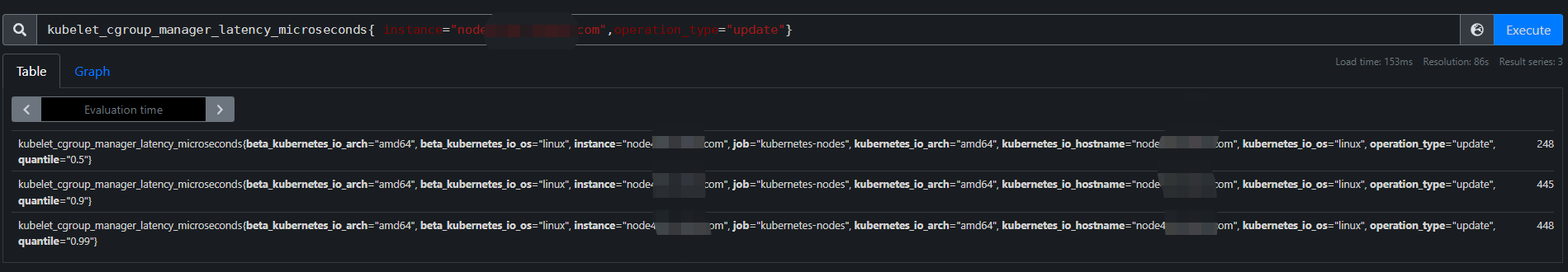Summary和Histogram都可以使用rate函数计算平均数

rate(kubelet_cgroup_manager_latency_microseconds_sum{ instance="node4.**.com",operation_type="update"}[10m]) / rate(kubelet_cgroup_manager_latency_microseconds_count{ instance="node4.**.com",operation_type="update"}[10m])


Histogram Summary

φ分位数和窗口范围 取决于histogram_quantile函数中φ 由客户端预先添加

HISTOGRAMS AND SUMMARIES

Prometheus metric_types

posted @ 2022-06-07 18:25  justtest1  阅读(543)  评论(0编辑  收藏  举报